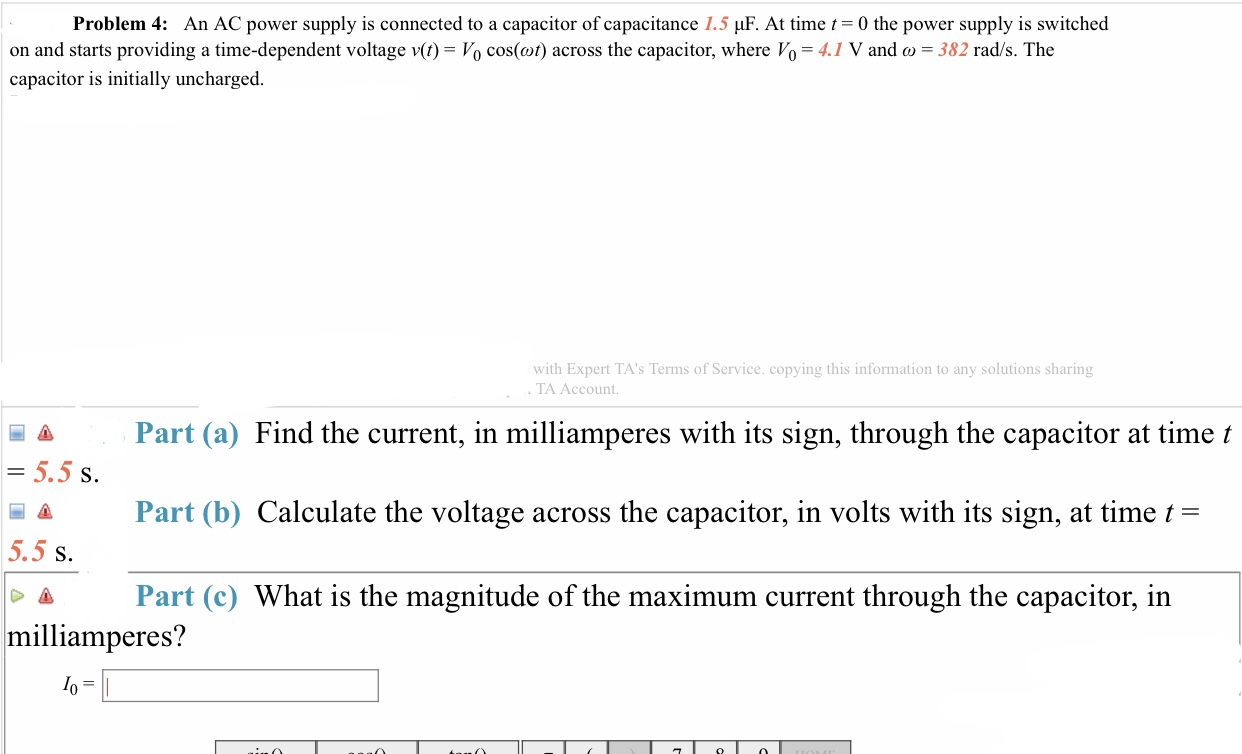Problem 4: An AC power supply is connected to a capacitor of capacitance 1.5 μF. At time0 the power supply is switchedon and starts providing a time-dependent voltage v(t)-Vo cos(a)) across the capacitor, where V0-41 V and ω-382 rad/s. Thecapacitor is initially unchargedwith Expert TA's Terms of Service. copying this information to any solutions sharingTA Account.A Part (a) Find the current, in milliamperes with its sign, through the capacitor at time tS.Part (b) Calculate the voltage across the capacitor, in volts with its sign, at time t-5.5 s.Δ Part (c) What is the magnitude of the maximum current through the capacitor, inmilliamperes?

Questionhelp_outlineImage TranscriptioncloseProblem 4: An AC power supply is connected to a capacitor of capacitance 1.5 μF. At time 0 the power supply is switched on and starts providing a time-dependent voltage v(t)-Vo cos(a)) across the capacitor, where V0-41 V and ω-382 rad/s. The capacitor is initially uncharged with Expert TA's Terms of Service. copying this information to any solutions sharing TA Account. A Part (a) Find the current, in milliamperes with its sign, through the capacitor at time t S. Part (b) Calculate the voltage across the capacitor, in volts with its sign, at time t- 5.5 s. Δ Part (c) What is the magnitude of the maximum current through the capacitor, in milliamperes? fullscreen
Step 1

a)

Current through the capacitor is,

Step 2

XC is the capacitive reactance.

Expression for XC is,

Step 3

Substituting the...

Want to see the full answer?

See Solution

Want to see this answer and more?

Our solutions are written by experts, many with advanced degrees, and available 24/7

See Solution
Tagged in

Alternating Current